Next: Operators Up: Fundamentals of Quantum Mechanics Previous: Expectation Values and Variances

Ehrenfest's Theorem

A simple way to calculate the expectation value of momentum is to evaluate the time derivative of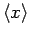, and then multiply by the mass: i.e.,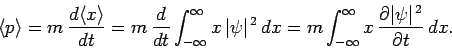(170)

However, it is easily demonstrated that(171)

[this is just the differential form of Eq. (154)], whereis the probability current defined in Eq. (155). Thus,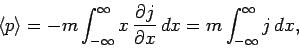(172)

where we have integrated by parts. It follows from Eq. (155) that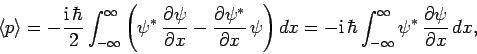(173)

where we have again integrated by parts. Hence, the expectation value of the momentum can be written(174)

It follows from the above that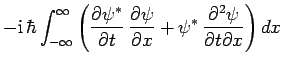(175)

where we have integrated by parts. Substituting from Schrödinger's equation (137), and simplifying, we obtain(176)

Integration by parts yields(177)

Hence, according to Eqs. (170) and (177),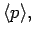(178)(179)

Evidently, the expectation values of displacement and momentum obey time evolution equations which are analogous to those of classical mechanics. This result is known as Ehrenfest's theorem.

Suppose that the potentialis slowly varying. In this case, we can expandas a Taylor series about. Keeping terms up to second order, we obtain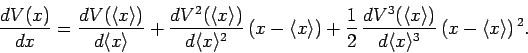(180)

Substitution of the above expansion into Eq. (179) yields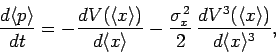(181)

since, and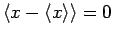, and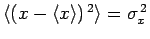. The final term on the right-hand side of the above equation can be neglected when the spatial extent of the particle wavefunction,, is much smaller than the variation length-scale of the potential. In this case, Eqs. (178) and (179) reduce to(182)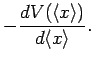(183)

These equations are exactly equivalent to the equations of classical mechanics, withplaying the role of the particle displacement. Of course, if the spatial extent of the wavefunction is negligible then a measurement ofis almost certain to yield a result which lies very close to. Hence, we conclude that quantum mechanics corresponds to classical mechanics in the limit that the spatial extent of the wavefunction (which is typically of order the de Boglie wavelength) is negligible. This is an important result, since we know that classical mechanics gives the correct answer in this limit.Next: Operators Up: Fundamentals of Quantum Mechanics Previous: Expectation Values and Variances
Richard Fitzpatrick 2010-07-20